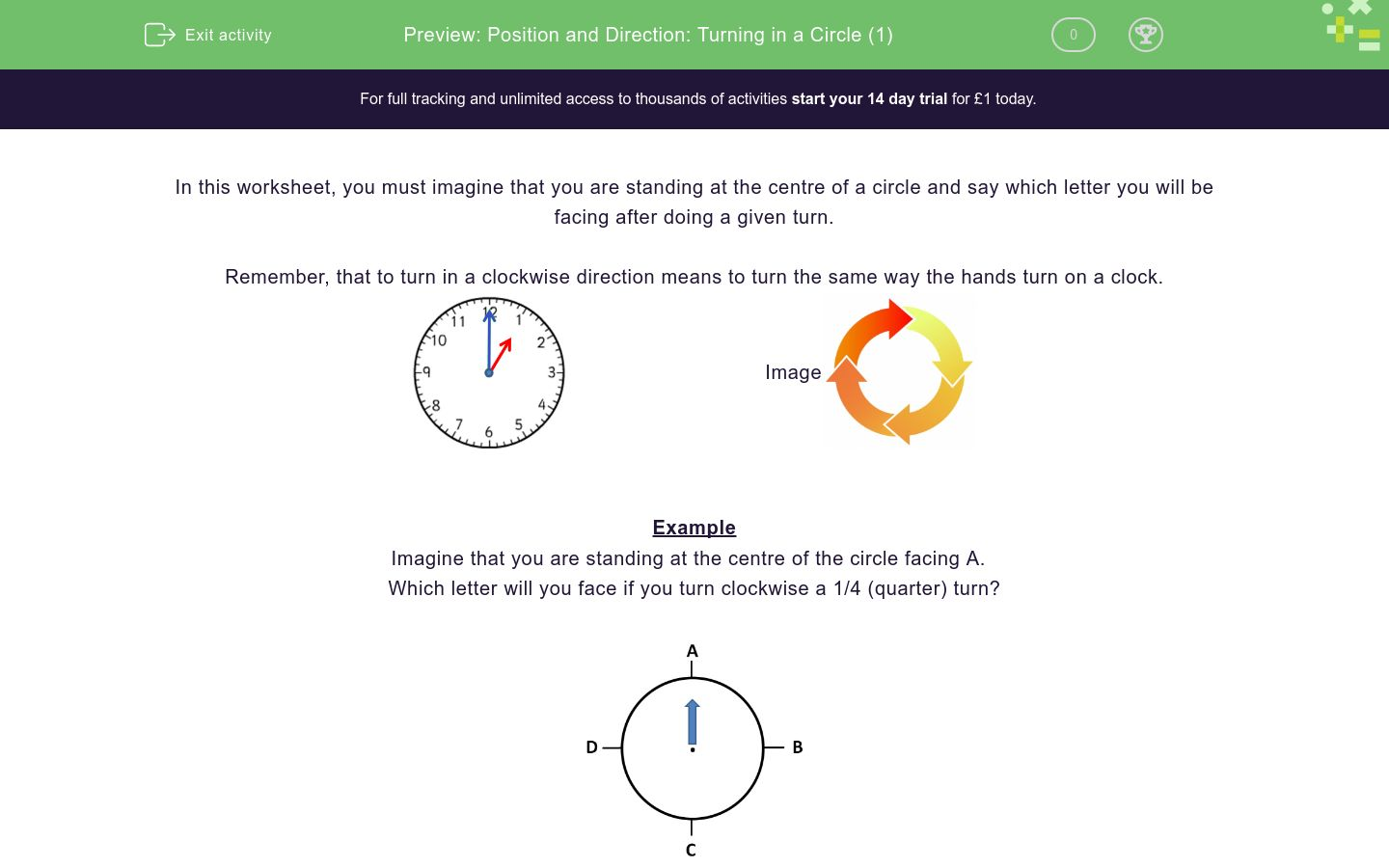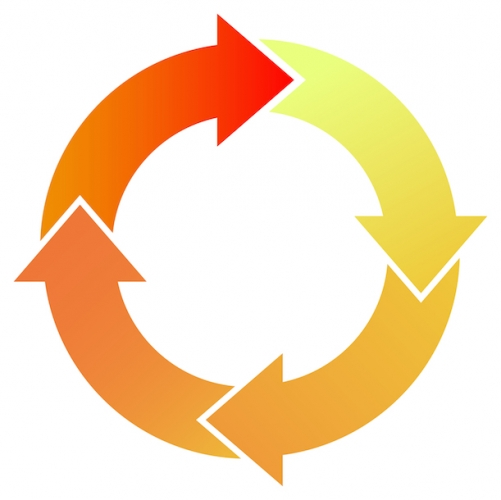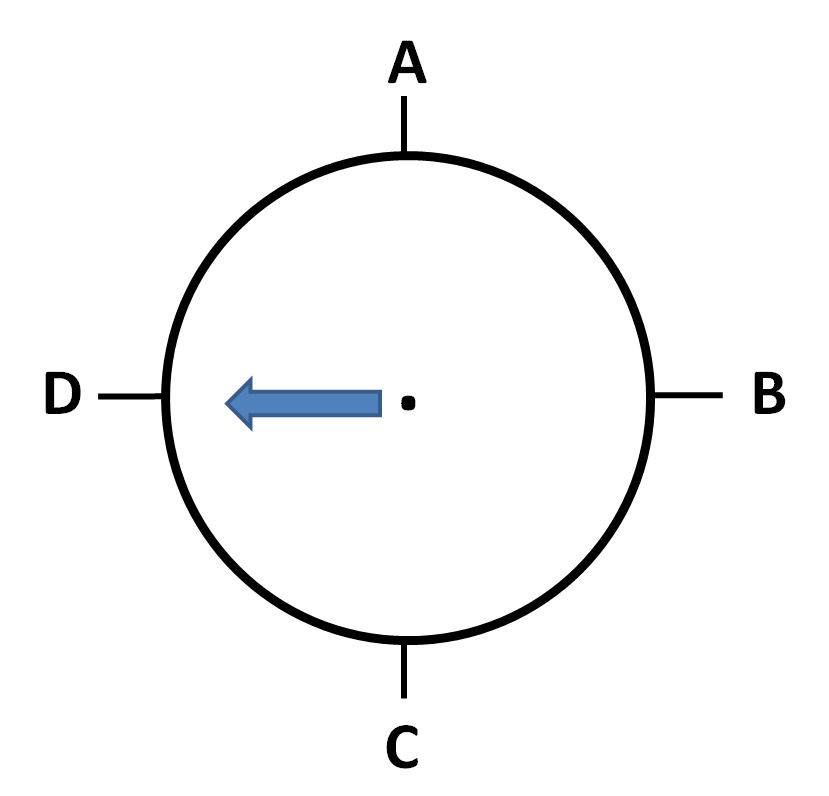# Position and Direction: Turning in a Circle (1)

In this worksheet, students give the result of quarter and half turns using a clockwise turn.Key stage:  KS 1

Curriculum topic:   Geometry: Position and Direction

Curriculum subtopic:   Use Language of Position/Direction

Difficulty level:### QUESTION 1 of 10

In this worksheet, you must imagine that you are standing at the centre of a circle and say which letter you will be facing after doing a given turn.

Remember, that to turn in a clockwise direction means to turn the same way the hands turn on a clock.Example

Imagine that you are standing at the centre of the circle facing A.

Which letter will you face if you turn clockwise a 1/4 (quarter) turn?Imagine that you are standing at the centre of this circle facing A.

Say which letter you will facing after doing the given turn.

A half turn (1/2) clockwise.A

B

C

D

Imagine that you are standing at the centre of this circle facing A.

Say which letter you will facing after doing the given turn.

A three-quarter (3/4) turn clockwise.A

B

C

D

Imagine that you are standing at the centre of this circle facing A.

Say which letter you will facing after doing the given turn.

A four-quarter (4/4) turn clockwise.A

B

C

D

Imagine that you are standing at the centre of this circle facing A.

Say which letter you will facing after doing the given turn.

A quarter turn (1/4) clockwise.A

B

C

D

Imagine that you are standing at the centre of this circle facing B.

Say which letter you will facing after doing the given turn.

A quarter turn (1/4) clockwise.A

B

C

D

Imagine that you are standing at the centre of this circle facing B.

Say which letter you will facing after doing the given turn.

A half turn (1/2) clockwise.A

B

C

D

Imagine that you are standing at the centre of this circle facing C.

Say which letter you will facing after doing the given turn.

A half turn (1/2) clockwise.A

B

C

D

Imagine that you are standing at the centre of this circle facing C.

Say which letter you will facing after doing the given turn.

A quarter turn (1/4) clockwise.A

B

C

D

Imagine that you are standing at the centre of this circle facing D.

Say which letter you will facing after doing the given turn.

A three-quarter (3/4) turn clockwise.A

B

C

D

Imagine that you are standing at the centre of this circle facing A.

Say which letter you will facing after doing the given turn.

A three-quarter (3/4) turn clockwise.A

B

C

D

• Question 1

Imagine that you are standing at the centre of this circle facing A.

Say which letter you will facing after doing the given turn.

A half turn (1/2) clockwise.C
EDDIE SAYS
That's right...a half turn (1/2) clockwise, moves you to letter C, opposite letter A.
• Question 2

Imagine that you are standing at the centre of this circle facing A.

Say which letter you will facing after doing the given turn.

A three-quarter (3/4) turn clockwise.D
EDDIE SAYS
Yes...you got it! A three quarter (3/4) turn clockwise moves you to face letter D. Always remember which way the hands of a clock turn, that's the same as clockwise.
• Question 3

Imagine that you are standing at the centre of this circle facing A.

Say which letter you will facing after doing the given turn.

A four-quarter (4/4) turn clockwise.A
EDDIE SAYS
Spot on! You have turned a whole turn (4/4) and are back where you started!
• Question 4

Imagine that you are standing at the centre of this circle facing A.

Say which letter you will facing after doing the given turn.

A quarter turn (1/4) clockwise.B
EDDIE SAYS
Well done! A one quarter (1/4) turn clockwise, takes you to letter B.
• Question 5

Imagine that you are standing at the centre of this circle facing B.

Say which letter you will facing after doing the given turn.

A quarter turn (1/4) clockwise.C
EDDIE SAYS
Excellent, even though we started at a different point, you have worked out that we have now moved a one quarter (1/4) turn to face letter C.
• Question 6

Imagine that you are standing at the centre of this circle facing B.

Say which letter you will facing after doing the given turn.

A half turn (1/2) clockwise.D
EDDIE SAYS
Great! A half turn (1/2) clockwise, moves you to face letter D.
• Question 7

Imagine that you are standing at the centre of this circle facing C.

Say which letter you will facing after doing the given turn.

A half turn (1/2) clockwise.A
EDDIE SAYS
Brilliant! A half turn (1/2) clockwise now means you are facing letter A.
• Question 8

Imagine that you are standing at the centre of this circle facing C.

Say which letter you will facing after doing the given turn.

A quarter turn (1/4) clockwise.D
EDDIE SAYS
Bingo! A one quarter (1/4) turn clockwise takes you to letter D.
• Question 9

Imagine that you are standing at the centre of this circle facing D.

Say which letter you will facing after doing the given turn.

A three-quarter (3/4) turn clockwise.C
EDDIE SAYS
Spot on! We have nearly turned a full circle to get to letter C.
• Question 10

Imagine that you are standing at the centre of this circle facing A.

Say which letter you will facing after doing the given turn.

A three-quarter (3/4) turn clockwise.D
EDDIE SAYS
Amazing! Even though the circle is tilted, you have spotted that a three quarter (3/4) turn moves you to letter D.
---- OR ----

Sign up for a £1 trial so you can track and measure your child's progress on this activity.

### What is EdPlace?

We're your National Curriculum aligned online education content provider helping each child succeed in English, maths and science from year 1 to GCSE. With an EdPlace account you’ll be able to track and measure progress, helping each child achieve their best. We build confidence and attainment by personalising each child’s learning at a level that suits them.

Get started